# Pinched CylinderThe purpose of this test is to check shell elements. You will use 2D meshes.Reference:

LINBERG G.M., OLSON M.D., New Developments in the Finite Element Analysis of Shells, COXP. Mech. Eng. and Nat. Aeronautical Establishment, National Research Council of Canada Vol. 4., 1969

##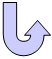Specifications

### Geometry Specifications

 Length: L = 600 m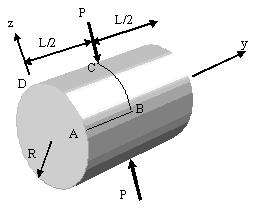Radius: R = 300 mm Thickness: th = 3 mm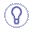For symmetry reasons, only 1/8 of the body is modeled. The given results are the same for 1/8 or for the whole body.

### Analysis Specifications

 Young Modulus (material): E = 3 MPa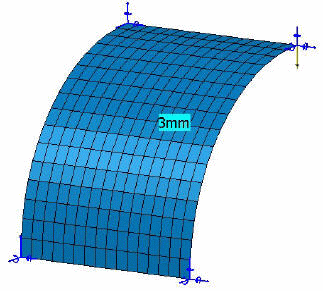Poisson's Ratio (material): ν = 0.3 Mesh Specifications: Imposed nodes on AB, BC, CD, DA (see the table of results for values) Restraints: On edge AB: Tz = Rx = Ry = 0 On edge BC: Ty = Rx = Rz = 0 On edge CD: Tx = Ry = Rz = 0 On edge DA: Tx = Ty = Ry = 0 Loads (Distributed force): F = 0.25 inward at C

##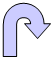Results

• The theoretical value of the radial displacement at the point load location is 18.25 mm.

• The results correspond to the radial displacement at point C.
The table below presents the analysis results.
Normalized results (computed results divided by analytical solution) are listed.

 Nodes Values Linear triangle (TR3) Parabolic triangle (TR6) Linear quadrangle (QD4) Parabolic quadrangle (QD8)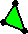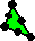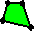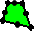Computed results  [mm] Normalized results Computed results  [mm] Normalized results Computed results  [mm] Normalized results Computed results  [mm] Normalized results 3 x 3 0.98 0.054 8.19 0.449 1.73 0.095 12.07 0.662 5 x 5 8.13 0.445 13.38 0.733 10.09 0.551 16.43 0.901 7 x 7 13.28 0.728 19.12 1.047 14.00 0.767 17.29 0.948 9 x 9 15.95 0.874 19.98 1.095 16.34 0.895 18.17 0.996 11 x 11 15.87 0.870 17.10 0.937 17.04 0.934 17.97 0.985 13 x 13 17.09 0.936 18.91 1.036 17.42 0.955 17.81 0.976 15 x 15 16.87 0.924 17.56 0.962 17.86 0.978 18.08 0.991 17 x 17 17.19 0.942 17.82 0.976 18.09 0.991 18.33 1.004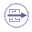To Perform the Test:

The pinched_cylinder_17nodes_qd8.CATAnalysis document presents a complete analysis of this case, computed with a mesh formed of parabolic quadrangle elements (QD8).

To compute the case with other types of elements and number of nodes, proceed as follow:

1. Open the CATAnalysis document.

2. In the Advanced Meshing Tools workbench, change the mesh specifications as indicated above.

3. Compute the case in the Generative Structural Analysis workbench.